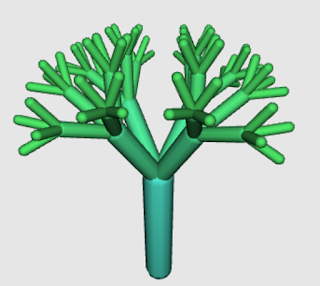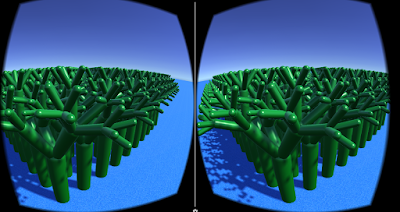## Sunday, July 09, 2017

### Create Object Multiples With a Script in Unity

It's not very hard to make a lot of some object in Unity, much easier than copying it many times. I used these instructions, Instantiating Prefabs at Runtime, to learn this, but I'm adding the twist that the item I'm multiplying is an imported Beetle Blocks 3D model.
Import Your Model. I'm importing this cool L-System tree by Eric Rosenbaum. See this post for more about importing 3D models from Beetle Blocks to Unity.Place that model in the game world and modify it however you want, scaling it, changing color, etc.Create an empty game object in the Hierarchy panel. Click Add Component and create a new C# script attached to it with this code (below). Call it what the object is, as I've called mine 'Trees'. It will appear in the Assets folder. Double click on it to edit the script. Your script title will be auto-created in the class name, i.e. public class Trees.  The class name MUST match the script name, so if you change one you have to change the other.

`````` using System.Collections;
using System.Collections.Generic;
using UnityEngine;
public class Trees : MonoBehaviour
{
public Transform model;
void Start ()
{
for (int z = 0; z < 10; z++) {
for (int x = 0; x < 10; x++) {
Instantiate (model, new Vector3 (x, 0, z), Quaternion.identity);
}
}
}
}
``````

The public Transform model variable will open a field in the GameObject inspector that you will fill in a moment.
Now create a new Prefab in the Assets folder (right click > Create > Prefab) and rename it to whatever your model is.
Drag your model from the Hierarchy pane onto the Prefab.
Now drag the prefab into the model field in the GameObject.
The models will not appear in the editing window. But run the emulator, and your many objects will appear!Do you want the trees more widely spaced? You can modify the script to take bigger steps:

```` for (int z = 0; z < 10; ``z+=3````) {
for (int x = 0; x < 10; ````x+=3````) {
Instantiate (model, new Vector3 (x, 0, z), Quaternion.identity);
}
}
``````Now you have many fewer trees because the upper limit is 10 meters total area. To get more increase that.

```` for (int z = 0; ``z < 30``; z+=3````) {
for (int x = 0; ````x < 30``; x+=3````) {
Instantiate (model, new Vector3 (x, 0, z), Quaternion.identity);
}
}
```````````` for (int i = 0; i < 100; i++) {